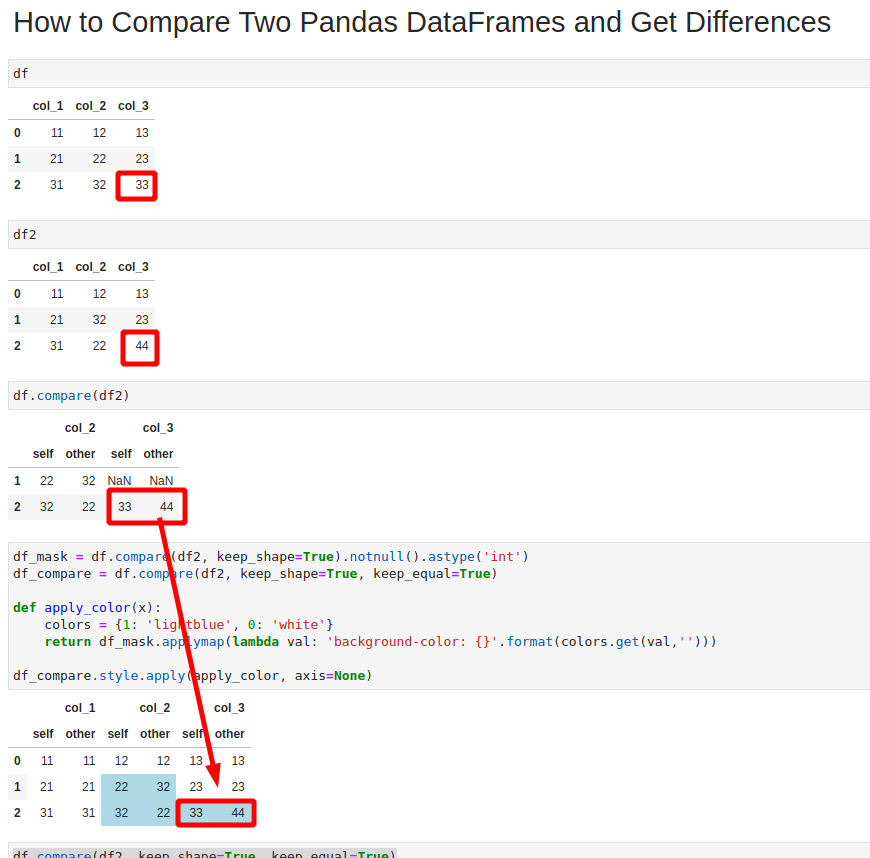## 1. Overview

In this tutorial, we're going to compare two Pandas DataFrames side by side and highlight the differences.

We'll first look into Pandas method `compare()` to find differences between values of two DataFrames, then we will cover some advanced techniques to highlight the values and finally how to compare stats of the DataFrames.

The image below show the final result:## 2. Setup

Let's have the next DataFrame created by the code below:

``````import pandas as pd
data = [('11','12','13'),
('21','22','23'),
('31','32','33')]
df = pd.DataFrame(data,
columns = ('col_1', 'col_2', 'col_3' ))
``````

DataFrame looks like:

col_1 col_2 col_3
0 11 12 13
1 21 22 23
2 31 32 33

Let's create second DataFrame by copying the first one and changing some values:

``````df2 = df.copy()
df2.iloc[1,1] = 32
df2.iloc[2,1] = 22
df2.iloc[2,2] = 44
``````

The second DataFrame looks like:

col_1 col_2 col_3
0 11 12 13
1 21 32 23
2 31 22 44

Can you find what are the differences just by watching both DataFrames? In next steps we will compare two DataFrames in Pandas.

## 3. Compare Two Pandas DataFrames to Get Differences

Pandas offers method: pandas.DataFrame.compare since version 1.1.0.

It gives the difference between two DataFrames - the method is executed on DataFrame and take another one as a parameter:

``````df.compare(df2)
``````

The default result is new DataFrame which has differences between both DataFrames. The new DataFrame has multi-index - first level is the column name, the second one are the values from the both DataFrames which are compared:

col_2 col_3
self other self other
1 22 32 NaN NaN
2 32 22 33 44

The method has several parameters which we will cover in next sections:

• `align_axis`
• `keep_shape`
• `keep_equal`

## 4. Compare Two Pandas DataFrames Side by Side - keeping all values

If you like to keep the original shape and all values (even if they are equal) then you can use - `keep_shape` and `keep_equal`.

Keep the original shape - all equal values are replaced by NaN values:

``````df.compare(df2, keep_shape=True)
``````
col_1 col_2 col_3
self other self other self other
0 NaN NaN NaN NaN NaN NaN
1 NaN NaN 22 32 NaN NaN
2 NaN NaN 32 22 33 44

Preserving the original values from both DataFrames can be done by both parameters:

``````df.compare(df2, keep_shape=True, keep_equal=True)
``````

The result is:

col_1 col_2 col_3
self other self other self other
0 11 11 12 12 13 13
1 21 21 22 32 23 23
2 31 31 32 22 33 44

## 5. Compare Two Pandas DataFrames Highlighting Differences

Finally let's cover how to highlight the difference between both DataFrames. Again we are going to use the method `compare()` with a combination of styling.

### 5.1. Compare and Highlight Difference between Two DataFrames

To compare two DataFrames get the difference and highlight them use the code below:

``````df_mask = df.compare(df2, keep_shape=True).notnull().astype('int')
df_compare = df.compare(df2, keep_shape=True, keep_equal=True)

def apply_color(x):
colors = {1: 'lightblue', 0: 'white'}

df_compare.style.apply(apply_color, axis=None)
``````

### 5.2. Explanation of the steps

First we are going to build a mask for our styling:

``````df_diff = df.compare(df2, keep_shape=True)

``````

col_1 col_2 col_3
self other self other self other
0 0 0 0 0 0 0
1 0 0 1 1 0 0
2 0 0 1 1 1 1

Next is to build comparison DataFrame:

``````df_compare = df.compare(df2, keep_shape=True, keep_equal=True)
df_compare
``````

the same as the previous step. The last thing to do is apply styling based on the mask to the comparison DataFrame:

``````def apply_color(x):
colors = {1: 'lightblue', 0: 'white'}

df_compare.style.apply(apply_color, axis=None)
``````

the final result is:

col_1 col_2 col_3
self other self other self other
0 11 11 12 12 13 13
1 21 21 22 32 23 23
2 31 31 32 22 33 44

## 6. Conclusion

To sum up, we looked at how to compare two DataFrames in Pandas side by side. We focused on finding differences, keeping the same shape and the equal values.

Finally we saw how to highlight the differences while showing DataFrames side by side.